### TOPIC 9 – COMPOUNDS OF METALS

VOLUMETRIC ANALYSIS
TOPIC 5: VOLUMETRIC ANALYSIS

The Concept of Volumetric Analysis
Explain the concept of volumetric analysis

Volumetric analysis is a quantitative analysis involving the measurement of different solutions. These solutions are made to react completely and the completion of the reaction is indicated by certain substances called indicators. The quantitative composition of the solution is then determined.

Important steps of volumetric analysis include:
• Weighing;
• Preparation of the solution;
• Titration; and
• Calculation

In volumetric analysis, we deal with volumes of solutions. That is why this quantitative determination of solutions of substances is called volumetric analysis.

The amount of a substance present in a solution is given in terms of its volume and its concentration. The volume of a solution is usually given in litres (dm3). The concentration of a solution is given in moles per litre (mol/dm3) or grams per litre (g/dm3).

Volumetric analysis is a means of finding the concentration of an unknown solution. For example, the concentration of an unknown solution of an acid can be found if it is reacted with a standard solution of an alkali. A standard solution is one whose concentration is well known and does not change with time.

In volumetric analysis, the reaction is carried out in a carefully controlled way. The volumes are measured accurately using a pipette and burette. The method is to add a solution of one reactant to the solution of another reactant until the reaction is complete. When the reaction is complete, we say the end-point has been reached. If the reactants are acids and bases, completion (end-point) is determined by the change in colour of an acid-base indicator. The method is called titration. In other reactions, completion is determined by a colour change of reactant(s). The concentration of one of the reactant solutions must be known in order to be able to find the concentration of unknown solution.

Significance of Volumetric Analysis
1. Volumetric analysis is used to quantify the amount of substances present in solutions by analytical procedure, which involves precise measurements of volumes of solutions and masses of solids.
2. Volumetric analysis helps in the determination of the accurate volumes and concentrations of the reacting substances, often solutions.
3. Volumetric analysis (titration) helps in the preparations of standard solutions.
4. Volumetric analysis knowledge helps in the standardization of acids and bases.

Volumetric Apparatus
Use volumetric apparatus

We have seen that volumetric analysis involves determinations of quantities of substances, usually acids and alkalis, present in volumes of solutions. This is usually done by using measuring apparatus.

Apparatus used in volumetric analysis is based on volume measurements and since the analysis demands high accuracy, the apparatus has to be calibrated with the highest possible accuracy. It is for this reason that all apparatus for volumetric analysis are specifically for this and not other purposes.

Apparatus used for volumetric analysis include, burette, pipette, burette stand, white tile, conical flask, filter funnel, reagent bottle, watch glass, beaker, measuring cylinder and measuring flask (or volumetric flask). For approximate measurements, measuring cylinders may be used. For accurate measurements of volumes, volumetric flasks are used.

Burette
This is a long glass tube with a narrow lower part, which is fitted with a tap that controls the amount of solution let out of the burette. This instrument is calibrated from 0 to 50 cm3.Before measuring the solution, rinse the burette with distilled water, then with the solution it is going to hold. It has to be filled to the tip and all gas bubbles removed. Thus, the burette is an apparatus used for transferring the solution to the titration vessel (normally a flask).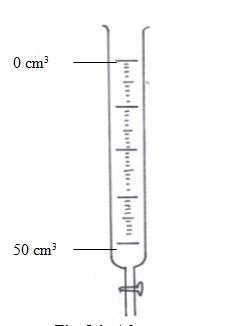Pipette
This apparatus has a wider middle part with narrow parts at either ends. The upper narrow part has a mark which marks the volume of all the space below it. If, say, the pipette is one that is marked 25 cm3, we can say that a solution, when filled in the pipette up to this mark, will have a volume of 25 cm3.

The pipette is used in transferring a standard solution to the titration flask. There are many types of pipettes depending on their volume capacity. The common ones are the 25-cmand 20-cm3 capacity pipettes. Less common ones are the 10-cm3 capacity.Before measuring the solution, rinse the pipette several times with distilled water and then with the solution to be measured; suck the rinsing solution above the graduated mark, then discard the rinsing.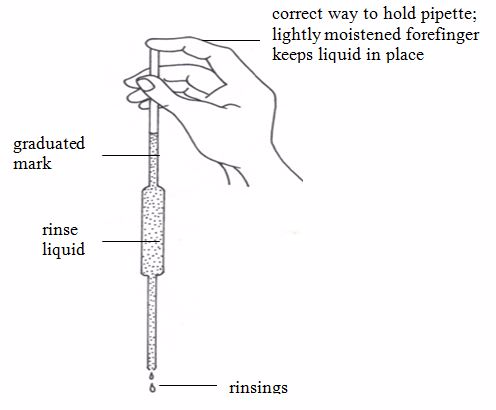The pipette is commonly filled by mouth suction but the use of pipette fillers is highly recommended. When using a pipette, never blow out the last drop.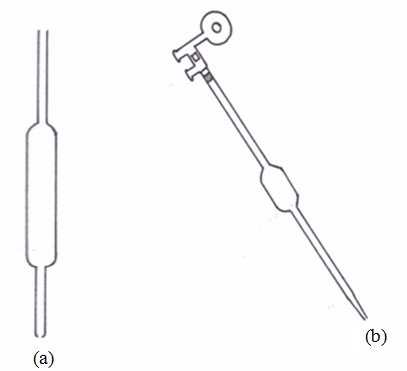(a) A pipette (b) A pipette and pipette filler (used to fill and empty pipettes)

The flask is made of glass and has a mark at the upper part of the narrow tube. The space in the flask up to this mark represents a certain volume. If a solution is filled up to this mark, the volume of the solution is equal to the volume indicated by inscriptions on the flask e.g. 50 cm3, 100 cm3, 150 cm3, 250 cm3, 500 cm3, etc.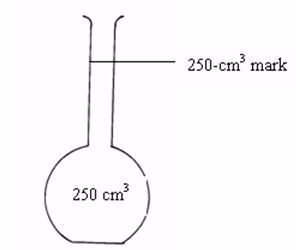Filter funnel
A filter funnel is required for effective transfer of the weighed solid, liquid or solution into the volumetric flask or burette.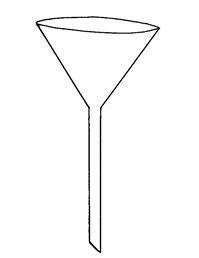A filter funnel

Wash bottle
Wash bottle contains water and when squeezed, water squarts out. This is used in washing down the remains of the weighed solid into the volumetric flask.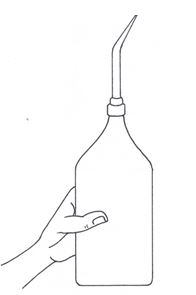A wash bottle

A weighing bottle
This is used in weighing the solute. It is a stoppered bottle. A watch glass can also be used to serve the same purpose.

Retort stand
A burette stand is used for holding the burette in place while carrying out volumetric analysis experiments.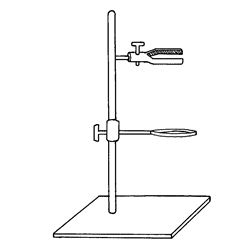A burette stand

Dropper
A dropper is used to add the indicator dropwise into the solution.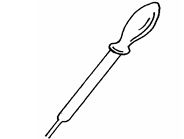White tile or paper
A white tile or piece of paper is placed under the flask to give a clear background for accurate observation of the colour change at the end of the reaction (end point).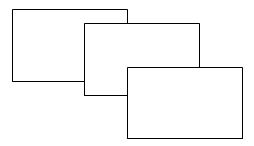The Steps for Preparation of Standard Solutions of Common Acids
Explain the steps for preparation of standard solutions of common acids

A standard solution is a solution of known concentration. For example, a solution containing 15g of sulphuric acid in 1 dm3 of solution is a standard solution.

It has now been approved that volumetric work should be based upon the molar (M) solution. A 1 molar (1M) solution of a compound is a solution which contains one mole of that compound in 1 dm3 of the solution. For example, 58.5g of sodium chloride (NaCl) dissolved in 1 dm3 of the solution makes a molar solution of sodium chloride (1M NaCl). Likewise, 106g of sodium carbonate (Na2CO3) in 1 dm3 of the solution gives a molar solution of sodium carbonate. Therefore, a 1 molar sodium carbonate solution contains 106g of the salt in 1 dm3 of the solution.

1 molar solution of some compounds commonly used in titration contain the following masses of the compounds in 1 dm3 of solution:
 Compound Relative molecular weight (1 mole) Sodium hydroxide, NaOH 40g Potassium hydroxide, KOH 56g Sulphuric acid, H2SO4 98g` Hydrochloric acid, HCl 36.5g Sodium carbonate, Na2CO3 106g Sodium bicarbonate, NaHCO3 84g

Derivative concentrations are also used e.g. 0.1M, 0.5M. 2M, etc.

Preparation of standard solutions
A standard solution is required as a starting point for volumetric analysis. We learned early that in order to find the unknown concentration of a substance in volumetric analysis, the concentration of one of the solutions must be known.

A small range of substances are suitable for direct preparation of accurately standard solutions. Substances that cannot be used for direct preparation of standard solutions include sodium hydroxide, potassium hydroxide and concentrated sulphuric acid. These substances absorb water vapour from the air and hence cannot be weighed out precisely without taking extra precautions. Apart from absorbing water vapour from the air, sodium and potassium hydroxides react with carbon dioxide of the air to form respective carbonates.

2NaOH(s) + CO2(g)→ Na2CO3(s) + H2O(l)

2KOH(s) + CO2(g)→ K2CO3(s) + H2O(l)

Some solutions are volatile in nature and so are likely to change slowly in concentration during ordinary use. These include concentrated hydrochloric acid and ammonia.

A compound commonly used for preparation of a precisely standard solution is anhydrous sodium carbonate. It is best prepared from highly pure sodium carbonate. This is achieved by heating sodium bicarbonate to constant mass to make sure the compound is fully decomposed.

2NaHCO 3(s) →Na2CO3(s) + H2O(g) + CO2(g)

The sodium carbonate so formed is suitable for preparation of a standard solution and can be weighed without undergoing any appreciable change in composition.

Precautions to be observed while preparing a standard solution
• Transference of the substance from the weighing bottle to the beaker or flasks should be done with outmost care so that not a single particle of the substance is lost.
• Undissolved substance should not be transferred to the measuring flask. Make sure all the solid dissolves into solution before transferring the solution to flask.
• During making up of the volume, the last drop of the water should be added carefully. Do not blow out the final drop.

Standard Solutions of Bases
Prepare standard solutions of bases

Preparation of 0.1M sodium carbonate solution
The molecular mass of sodium carbonate (Na2CO3) is 106g. Therefore, a molar (1M) solution of sodium carbonate contains 106g in 1 dm3 (1000 cm3) of solution.

In order to prepare 0.1M solution of the carbonate, we have to weigh 10.6g of the carbonate and put it into a volumetric flask, which has a capacity of 1000 cm3.

However, normally 250 cm3 flasks are used. This means, in a 250 cm3 flask we have to add 10.6/4= 2.65g calcium of sodium carbonate.

Thus, 1000 cm3 ≡ 10.6g

250 cm3 ≡ X g

X = 250/1000×10.6 = 10.6/4 = 2.65g

The same procedure can be followed when preparing 0.25M, 0.5M, 2M, etc. of the solutions.

Procedure
• Weigh exactly 2.65g of sodium carbonate using a common balance and put it onto a watch glass.
• Transfer it slowly into a beaker of 500-cm3 capacity containing about 50 cm3 of hot distilled water.
• Wash down the watch glass with a jet of hot distilled water from a wash bottle and allow the washings to fall into the beaker (figure 5.7). Make sure all the sodium carbonate is washed into the beaker.
• Stir with a glass rod until all the solid is completely dissolved, and then cool the solution to room temperature. Leave the rod standing in the solution.
• Pour the solution carefully down the glass rod into a 250 cm3 measuring flask.
• Wash the beaker out at least twice with jets of cold distilled water directed round the slides and pour the washings down the glass rod into the measuring flask (figure 5.8).
• Shake the flask gently and fill it up with cold distilled water almost to the mark.
• Add more distilled water drop by drop from a pipette until the meniscus is on the graduation mark (figure 5.9).
• Stopper the measuring flask and shake well. The liquid should then be exactly 0.1M sodium carbonate solution.

Preparation of standard solutions of other bases of different molarities e.g. 0.2M, 0.5M, 1.0M, 2.0M, etc. can be achieved by using the above procedures. The only variable will be the weight of the solids and volume of water as stated early.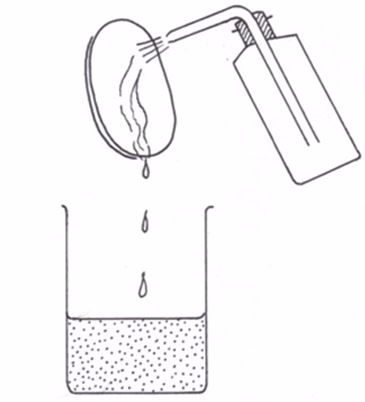Washing the watch glass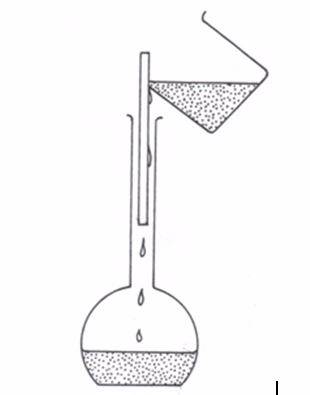Filling the washings into the flask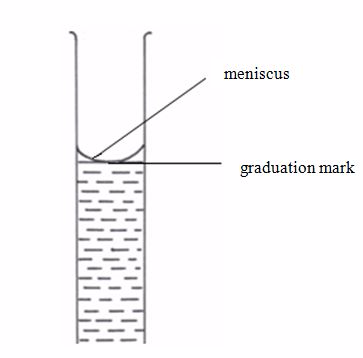Correct reading of the liquid volume

Acid-base Titration Experiments
Carry out acid-base titration experiments

Preparation of 0.1M sulphuric acid solution
A standard solution of sulphuric acid cannot be prepared directly because concentrated sulphuric acid is hygroscopic in nature (it tends to absorb water vapour from the air diluting itself) and is never reliably pure. A solution a little above 0.1M is prepared and then standardized and diluted with distilled water to exactly 0.1M.A molar solution of H2SO4 contains 98g of pure acid in 1 dm3. Therefore, the 0.1M acid contains 9.8g of the acid in 1 dm3 of the solution. The pure concentrated acid has a density (concentration) of about 1.8g/cm3. So, 9.8g of it occupy about 9.8/1.8 = 5.5cm3

The preparation of a standard solution of sulphuric acid involves two stages:
1. Diluting a concentrated solution of the acid to an approximate molarity.
2. Finding the exact concentration of the acid (standardizing it) by titrating it against a standard solution of a base (previously prepared).

Dilution of concentrated sulphuric acid
Caution: Make sure you wear safety goggles and gloves before carrying out this experiment.

Procedure
• Cautiously, because the acid is very corrosive, take 5.5 – 6.0 cm3 of concentrated sulphuric acid in a small measuring cylinder.
• Pour the acid carefully, with stirring, into a 250-cm3 volumetric flask containing about 100 cm3 of cold distilled water.
• Pour this solution into, say, 700cm3 of cold distilled water in a measuring flask of capacity 1000cm3.
• Wash out the acid solution remaining in the measuring cylinder with cold distilled water twice and add the washings into the measuring flask.
• Then add distilled water approximately to the mark on the measuring flask, stopper it, and shake well.

This should give sulphuric acid of concentration a little above 0.1M. The diluted acid is now standardized with the 0.1M sodium carbonate solution prepared above.

Determination of the molarity (standardization) of sulphuric acid solution by titrating it against 0.1M sodium carbonate solution
The estimation of the concentration of a solution of an acid by reacting the acid with a standard alkali solution is known as titration. The end-point of an acid-base reaction is commonly determined by using a substance known as an indicator.

Procedure
• Measure 25 cm3 of 0.1M sodium carbonate solution and transfer it into a conical flask, using a pipette. Add a few drops of methyl orange indicator. This will turn the sodium carbonate solution yellow.
• Set up the apparatus as shown in figure 5.10
• Pour the acid into a 50-cm3 burette. Read and note the level of the acid in the burette.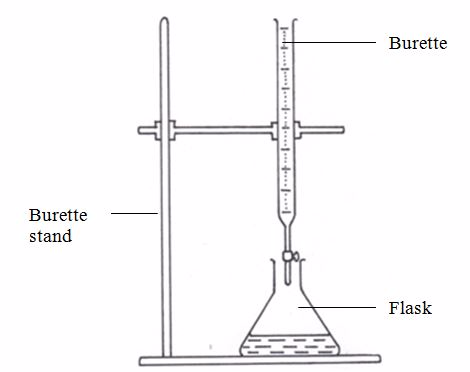Titration setup
• By means of a tap at the base of the burette, drip the acid slowly into the conical flask, swirling the flask continuously until the colour of the liquid in the flask turns orange. This is the end-point of titration. Record the new level of acid in the burette.
• Repeat the titration three to four times, noting the initial and final reading of the burette each time.
• Find the volume of the acid as shown below:
 Titration Rough titration (Pilot) Titre 1 Titre 2 Final burette reading 24.20 23.65 23.55 Initial burette reading 0.00 0.00 0.00 Volume of acid added 24.20 23.65 23.55

Neglecting the first (rough) trial run, the average titration is 23.60 cm3

Calculation
The first step in calculating the molarity of any solution from the results of an acid-base titration is to write the equation for the reaction. From the equation, find the number of reacting moles of the acid and base.

Na2CO3(aq)1 mole+ H2SO4(aq)1 moleNa2SO4(aq) + CO2(aq) + H2O(l)

Now we have the following data.
• Volume of acid, Va = 23.60 cm3
• Volume of base, Vb = 25.00 cm3 (this is the average amount of the base that was added to the flask in titration)
• Molarity of acid, Ma = ?
• Molarity of base, Mb = 0.1M
• Number of moles of acid, Na = 1
• Number of moles of base, Nb = 1

The molarity of the acid can be calculated from the following general formula: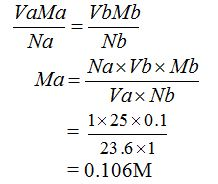The concentration of sulphuric acid is 0.106M.

To make the molar concentration of the sulphuric acid solution exactly equal to that of the sodium carbonate solution (0.1M), 23.6 cm3of the acid must be diluted to 25 cm3, that is, 1.4 cm3of distilled water must be added to 23.6 cm3of the acid.

Remember it was stated early that in order to prepare 0.1M sulphuric acid solution, you need to dissolve 9.8g of the acid in 1000 cm3 (1 dm3) of distilled water. Assume that some of the acid was wasted through spillage and mishandling and that only 920 cm3 of the acid was left. If, say, 920 cm3 of the acid was left, it can be made exactly 0.1M by the addition of 920×1.4/23.6 = 55cm3of distilled water. This gives exactly 0.1M of the acid. This is the same as saying that, if 23.6 cm3 of the acid were diluted with 1.4cm3 of distilled water, then 920cm3 of the acid would be diluted with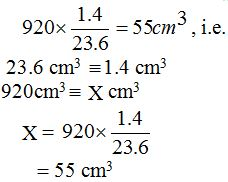If, for instance, the volume of acid left was, say, 850 cm3, the amount of distilled water to be added would be = 850×1.4/23.6 = 50.4cm3. This, also, would give exactly 0.1M of the acid.

In principle, the amount of distilled water to be added is always calculated based on the amount of the acid left as exemplified above.

These two standard alkaline and acidic solutions can be used to standardize other solutions, e.g. sodium hydroxide, potassium hydroxide, hydrochloric acid, nitric acid, etc. You may dilute any base or commercial acid to some required concentration e.g. 0.2M, 0.5M, 0.25M, etc and then standardize it by similar procedures.

Choice of indicators in acid-base titration
We learned early that the estimation of the concentration of a solution of an acid or base by reaction with a standard alkali or acid solution respectively, is known as titration. The end-point of an acid-base titration is commonly determined using substances known as indicators, which usually portray certain characteristic colours when in alkaline or acid solutions.

The indicators in acid-base titrations must be chosen carefully because the choice of an inappropriate indicator would lead to an incorrect result. The choice of an indicator is based on the strength of an acid or base involved in the reaction.

There are three common indicators which are used in titration experiments involving acids and bases namely, methyl orange, litmus and phenolphthalein. The other indicators in less common use are as included in the table below. The table shows the colours which each of these indicators take up in acid or alkaline solution.
Colour of indicators in acid and alkaline solutions
 Indicator Colour of indicator acid solution alkaline solution Methyl orange pink yellow Litmus red blue Phenolphthalein colourless pink Malachite green yellow blue/green Thymol blue red yellow Bromocresol green yellow blue Bromothymol blue yellow blue

Indicators suitable for particular types of acid-base reactions are as given in the table below:
Indicators suitable for different acid-base reactions
 Acid-base titration Example Choice of indicator Strong acid/strong base H2SO4 and NaOH Any indicator Weak acid/strong base CH3COOH (ethanoic acid) and KOH Phenolphthalein Strong acid/weak base HCl and NH3 Methyl orange Weak acid/weak base CH3COOH and NH3 No satisfactory indicator available

Common Mineral Acids
Standardize common mineral acids

Data for calculations of volumetric analysis problems are obtained from volumetric analysis experiments. For any volumetric analysis problem, at least one standard solution is required. A correctly balanced reaction equation (from which moles ratios can be derived) is a prerequisite for all these calculations. This is because the mole ratio is an integral part of the general expressionused for all volumetric analysis calculations. The general expression is given by: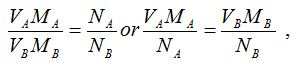where;
• VA = Volume of acid
• MA = Molarity of acid
• VB = Volume of base
• MB = Molarity of base
• NA = Number of moles of acid
• NB = Number of moles of base

The Relative Atomic Mass of Unknown Element in an Acid or Alkali
Find the relative atomic mass of unknown element in an acid or alkali

Example 1

12.5 cm3 of 0.5M sulphuric acid neutralized 50 cm3 of a given solution of sodium hydroxide. What is the molarity of the alkali?

Solution

Reaction equation is:

H2SO4(aq) + 2NaOH(aq) →Na2SO4(aq) + 2H2O(l)

From the equation, 1 mole of sulphuric acid solution reacts with 2 moles of sodium hydroxide solution. So, the number of moles of the acid, NA = 1 and the number of moles of base, NB = 2.The other data are as follows:

VA = 12.5 cm3, MA = 0.5M and VB = 50 cm3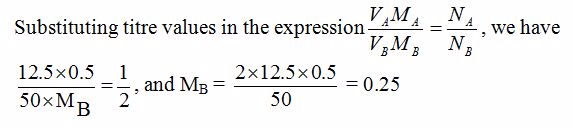So, the molarity of the acid = 0.25M

Example 2

20 cm3 of a solution containing 7g/dm3 of a metal hydroxide, XOH, were exactly neutralized with 25 cm3 of 0.10M hydrochloric acid.
1. Write a balanced chemical equation for the neutralization of the metal hydroxide, XOH, with hydrochloric acid.
2. Calculate the concentration of the metal hydroxide in moles per dm3.
3. (i) Calculate the molar mass of XOH (ii) Identify element X

Solution

<!–[endif]–>HCl(aq) + XOH(aq)→ XCl(aq) + H2O(l)

From the reaction equation, NA = 1 and NB = 1.

VA = 25 cm3, VB = 20 cm3, MA = 0.1M, MB = ?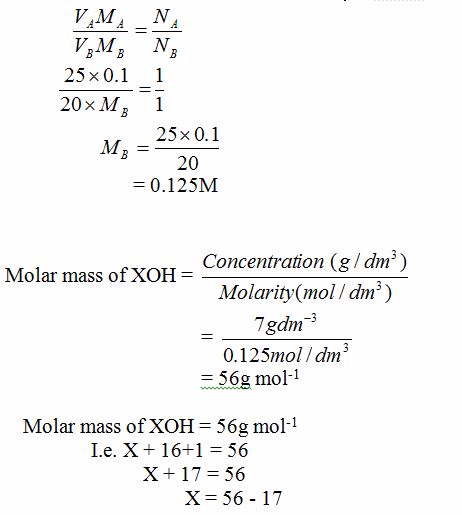X = 39g

Therefore, element X is potassium (K)

The Percentage Purity of an Acid or an Alkali
Calculate the percentage purity of an acid or an alkali

Example 3

5.1g of impure sodium carbonate solution was dissolved in water to make 500 cm3 of solution. 20 cm3 of this solution was titrated against 20.45 cm3 of 0.04M hydrochloric acid. Calculate the percentage purity of the sodium carbonate solid.

Solution

To calculate the concentration of impure Na2CO3 solution:5.1g per 500 cm3 = 10.2g dm-3Reaction equation is:

Na2CO3(aq) + 2HCl(aq) → 2NaCl(aq) + H2O(l) + CO2(g)

From the equation, NA =2 and NB = 1.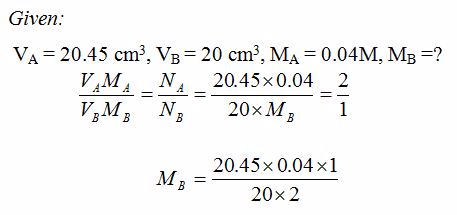= 0.02045M

Therefore, molarity of the base = 0.02045M

To calculate the concentration of pure base (Na2CO3 solution) in g dm-3:

Concentration = Molarity × Molar mass

But molar mass of Na2CO3 = 106.

So, concentration (g/dm3) = 0.02045 × 106 = 2.1677g dm-3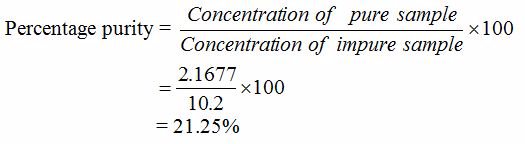The percentage purity of sodium carbonate = 21.25%

The Number of Molecules of Water of Crystallization of a Substance
Find the number of molecules of water of crystallization of a substance

Example 4
0.465g of a hydrated form of sodium carbonate exactly reacts with 75 cm3 of 0.10M hydrochloric acid. Calculate the number of molecules of water of crystallization present in one mole of the hydrated salt.
Solution

The balanced reaction equation for the anhydrous salt is:

Na2CO3(s) + 2HCl(aq) → 2NaCl(aq) + CO2(g) + H2O(l)

Number of moles of HCl present in 75 cm3 = 75/1000×0.1 = 0.0075M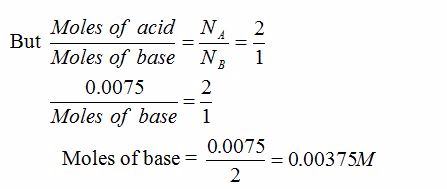Mass of 0.00375 moles of Na2CO3 = 0.00375 × 106 = 0.3975g (= mass of anhydrous salt)

Molar mass of anhydrous salt = 106g

Mass of water contained in the hydrated salt = mass of hydrated salt – mass of anhydrous salt

= 0.465 – 0.3975

= 0.0675g

Therefore, the mass of water of crystallization = 0.0675g

But, remember that mass of the anhydrous salt = 0.3975g

Now, let the formula of the hydrated salt be Na2CO3.XH2O, where X is the number of moles of water crystallization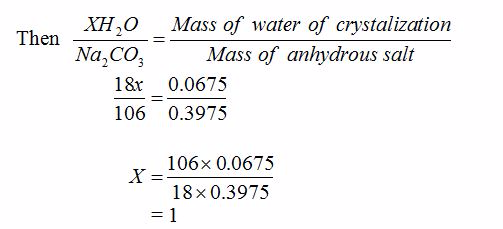Therefore, the formula of the salt is Na2CO3.H2O

The Application of Volumetric Analysis in Real Life Situations
Explain the application of volumetric analysis in real life situations

Volumetric analysis has a variety of laboratory and industrial applications in everyday life. The following are just a few of the applications (uses) of volumetric analysis in daily life:
• Use in preparation of standard solutions: Standard solutions are prepared by applying the knowledge of volumetric analysis. Volumetric analysis is used in school, college and university chemistry laboratories to determine concentrations of unknown substances. The titrant (the known solution) is added to a known quantity of analyte (unknown solution) and a reaction takes place. Knowing the volume of the titrant allows one to determine the concentration of the unknown substance.
• Use in environmental and water safety: Titration is important in environmental chemistry, where scientists can use it to analyze acid rain or contaminants in surface water samples. Environmental studies usually involve an analysis of precipitation and its response to pollution. To quantify the degree of contamination in natural rainwater or snow, titration is used. The process is quick and results are reliable. Since most titration processes do not require expensive or specialized equipment, the test can be performed often and in different areas with relatively little effort.The safety of water is based on its chemical ingredients. By analyzing wastewater, the extent of contamination and the requirements for filtering and cleaning can be determined. Titration is a key mechanism in this analysis. Often, more specialized titration equipment is used in this application, which measures ammonia levels in combination with other reactants to quantify other chemicals present.
• Use in food and beverage industry: In the food and beverage industry, manufacturers must ensure their products meet certain quality criteria or contain standard concentrations of specific additives, so titration is often used to analyze the products before sale. Wine is often affected by its degree of acidity. It is possible to improve wine production by measuring acidity using titration. Simple, inexpensive titration kits are available to winemakers for this purpose. The results of a titration test on wine can suggest if additional ingredients are necessary to maintain its quality.In general, all brewing industries and distilleries apply the knowledge of volumetric analysis (titration) to determine the acidity and alcohol contents of their beers and other alcoholic beverages.The process also finds ample use in food industry. The compounds which make up food products help determine their nutritional implications. Titration is one technique that assists in these studies. The acidity of orange juice, for example, is easily determined using a standard titration process. In this process, an electrode is added to a solution made up of orange juice and deionized water. The titrant catalyst then measures the acidity of the juice. Manufacturers can use the technique to vary this quality to satisfy customers or those with special nutritional needs.
• Use in agriculture: Volumetric analysis technique is used to determine the soil pH. This is important because, if the pH of a certain soil is found to be extremely low or high, corrective measures are taken by adding the correct quantity of agricultural limes or other chemicals to make the soil suitable for plant growth.The method is also used by agronomists and farmers to analyse the kind and amount of plant nutrient elements present in a particular sample of soil, the knowledge of which helps determine soil fertility.

Industrial and Laboratory Skills of Volumetric Analysis
Compare industrial and laboratory skills of volumetric analysis

The knowledge of volumetric analysis (titration) is used in hospitals and medical laboratories to carry out such duties as preparation of solutions and suspensions, blood analysis, and diagnosis of certain diseases and health problems. For example, when dissolving a solid drug to make a solution for injection, utmost precision is required to measure the correct volume of liquid to be used to dissolve a correct amount of solid drug to prepare the solution of a given concentration to inject to a patient.

Also titration is very important in the pharmaceutical industry, where precise measurements of quantities and concentrations are essential throughout the manufacturing process. Titration is thus an important part of the pharmaceutical industry to ensure quality control. Many variations of the titration technique are used, and specialized equipment for pharmaceutical titration is often developed to make the process more efficient.

Select the fields to be shown. Others will be hidden. Drag and drop to rearrange the order.
• Image
• SKU
• Rating
• Price
• Stock
• Availability